Function Repository Resource:

# NewtonMethod

Approximate the root of a function using Newton’s method

Contributed by: Dennis M Schneider
 ResourceFunction["NewtonMethod"][f,{x,x0},n] returns the root approximation obtained by applying Newton’s method at most n times to a differentiable function f with starting value x0.

## Examples

### Basic Examples (3)

Use Newton’s method to approximate the root of a function:

 In:=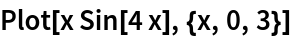Out=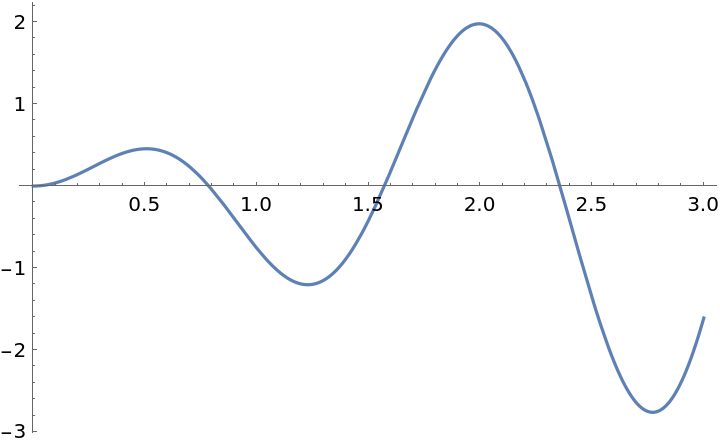Approximate the root around x=1.5:

 In:=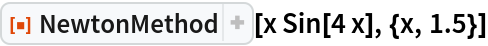Out=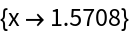Show the iterations found:

 In:=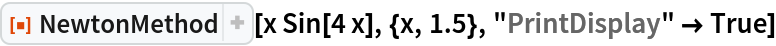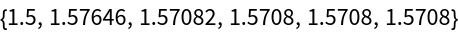Out=### Scope (2)

A more complicated function. First, plot it:

 In:=Out=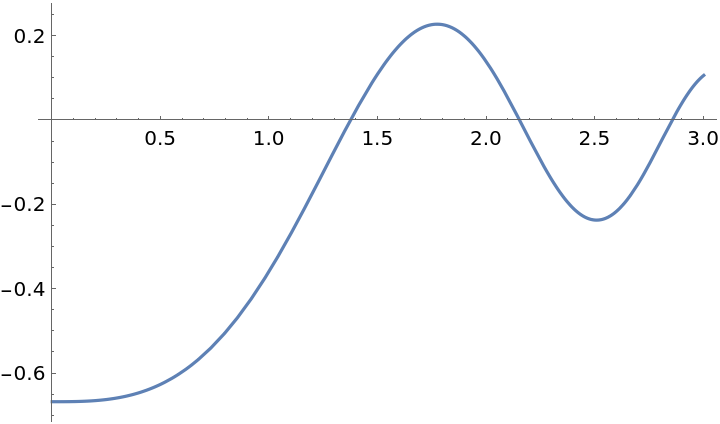Approximate the root around x=1:

 In:=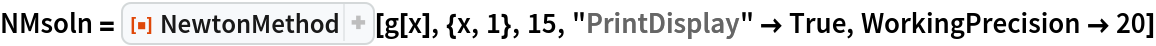Out=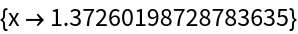Compare with FindRoot:

 In:=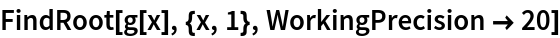Out=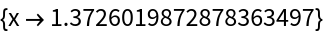Another complicated function:

 In:=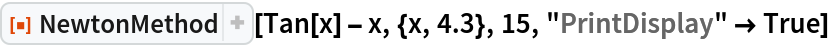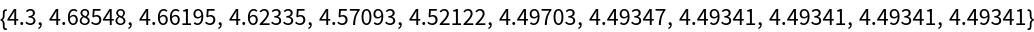Out=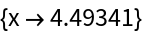Compare with FindRoot:

 In:=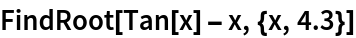Out=## Publisher

Dennis M Schneider

## Version History

• 1.0.0 – 20 August 2019